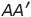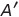Select Page

# Three Dimensional Geometry Maths 12 Science CBSE Solutions for MCQ in English

Three Dimensional Geometry Maths 12 Science CBSE Solutions for MCQ in English to enable students to get Solutions in a narrative video format for the specific question.

Expert Teacher provides Three Dimensional Geometry Maths 12 Science CBSE Solutions for MCQ through Video Solutions in English language. This video solution will be useful for students to understand how to write an answer in exam in order to score more marks. This teacher uses a narrative style for a question from Three Dimensional Geometry not only to explain the proper method of answering question, but deriving right answer too.

Please find the question below and view the Solution in a narrative video format.

Question:

Solution Video in English:

## Similar Questions from CBSE, 12th Science, Maths, Three Dimensional Geometry

Question 1 : If the cartesian equations of a line arewrite the vector equation for the line. (View Answer Video)

Question 2 : Find the distance of the plane : 3x - 4y + 12z = 3 from the origin. (View Answer Video)

Question 3 : If linesandintersect, then find the value of k and hence find the equation of plane containing these lines. (View Answer Video)

Question 4 : Find the angle between the lines whose direction ratios are a, b, c and b - c, c - a, a - b. (View Answer Video)

Question 5 : Find the distance of the point (-1, -5, -10) from the point of intersection of the lineand the plane(View Answer Video)

### Matrices

Question 1 : If,find the value of y.  (View Answer Video)

Question 2 : Find the value of z,  from the equation:(View Answer Video)

Question 3 : Find the value of Y, ifand. (View Answer Video)

Question 4 : Compute:. (View Answer Video)

Question 5 : If, write, whereis the transpose of A? (View Answer Video)

### Inverse Trigonometric Functions

Question 1 : IfFind the values of x. (View Answer Video)

Question 2 : Write in the simplest form:(View Answer Video)

Question 3 : What is principal value of(View Answer Video)

Question 4 : Write the principal value of(View Answer Video)

Question 5 : Write in the simplest form:(View Answer Video)

### Application of Derivatives

Question 1 : Sand is pouring from a pipe at the rate of. The falling sand forms a cone on the ground in such a way that the height of the cone is always one-sixth of the radius of the base. How fast is the height of the sand cone increasing, when the height is 4 cm? (View Answer Video)

Question 2 : The slope of the tangent to the curve:Question 3 : A tank with a rectangular base and rectangular sides, open at the top is to be constructed so that its depth is 2m and volume isIf the building of tank costs Rs.70 per sq meter for the base and Rs.45 per sq meter for sides. What is the cost of least expensive? (View Answer Video)
Question 4 : For the curve, y=if x increases at the rate of 2 units/sec, then how fast is the slope of curve changing when x=3? (View Answer Video)
Question 5 : The line y = mx + 1 is a tangent to the curveif the value of m is: (View Answer Video)